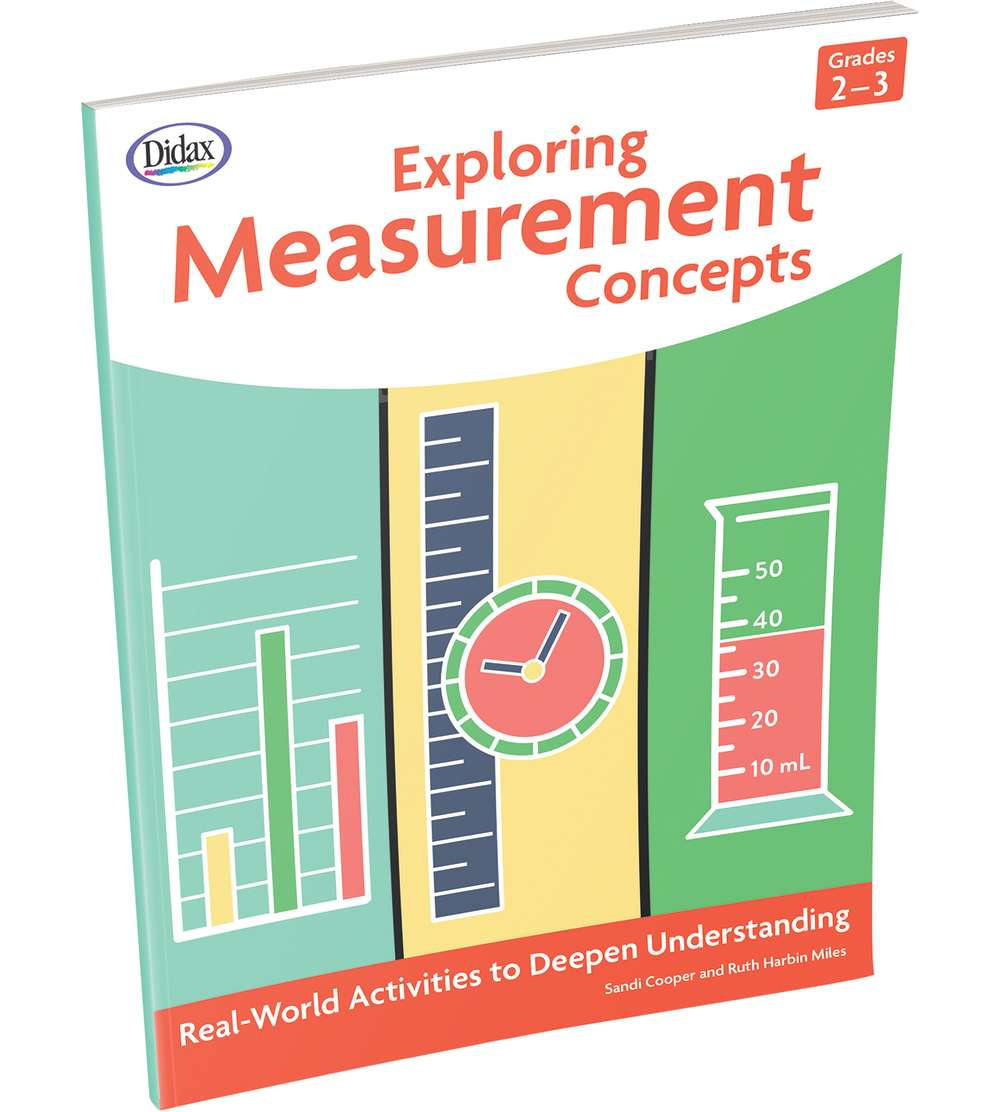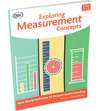•# Exploring Measurement Concepts, Grades 2-3

Product Number: DD-211731
You must be logged
\$14.99
This resource features more than 40 classroom-tested activities for exploring measurement. Students will explore length using...
Quantity

This resource features more than 40 classroom-tested activities for exploring measurement. Students will explore length using rulers and yardsticks; mass and volume using scales and liters; and area and perimeter using color tiles and graph paper.

In keeping with the current math standards, students are asked to:
• Estimate and compare lengths in standard units
• Estimate and measure the mass and volume of classroom objects
• Select and use appropriate measurement tools
• Solve word problems involving length, distance, mass and volume
• Tell and write time to nearest minute using a.m. and p.m.
• Generate measurement data and display in graphs and line plots
• Calculate area and perimeter, relating area to addition and multiplication
These can be used as small-group work or whole-class activities.
DD-211731